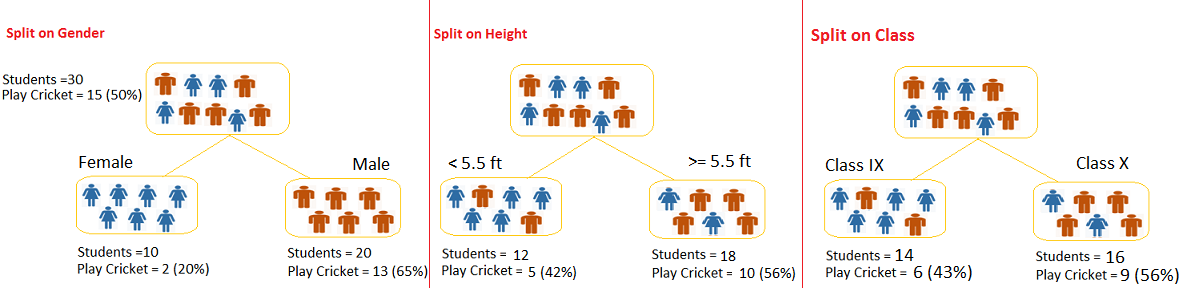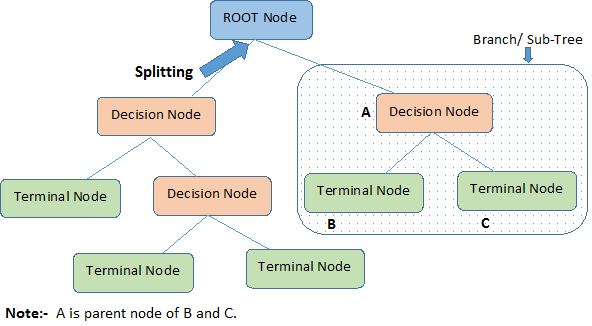Home » Decision Tree – Simplified!

# Decision Tree – Simplified!

I started working as a business analyst in my previous organisation. I transitioned from a Business Intelligence (BI) Analyst to become a Business Analyst. During the initial days of tenure as a business analyst, I had a bias towards using a classification technique – DECISION TREE. This was because of its inherent simplicity and many advantages. We will discuss these in more details later in this article.

Later on, I figured out that decision trees are one of the most commonly used technique among all business analysts. It can not only help us with prediction and classification, but also is a very effective tool to understand the behavior of various variables. In this article, we will discuss this algorithm in detail.

### What is a Decision Tree?

Decision tree is a type of supervised learning algorithm (having a pre-defined target variable) that is mostly used in classification problems. It works for both categorical and continuous input and output variables. In this technique, we split the population or sample into two or more homogeneous sets (or sub-populations) based on most significant splitter / differentiator in input variables.Example:-

Let’s say we have a sample of 30 students with three variables Gender (Boy/ Girl), Class( IX/ X) and Height (5 to 6 ft). 15 out of these 30 play cricket in leisure time. Now, I want to create a model to predict who will play cricket during leisure period? In this problem, we need to segregate students who play cricket in their leisure time based on highly significant input variable among all three.

This is where decision tree helps, it will segregate the students based on all values of three variable and identify the variable, which creates the best homogeneous sets of students (which are heterogeneous to each other). In the snapshot below, you can see that variable Gender is able to identify best homogeneous sets compared to the other two variables.As mentioned above, decision tree identifies the most significant variable and it’s value that gives best homogeneous sets of population. Now the question which arises is, how does it identify the variable and the split? To do this, decision tree uses various algorithms, which we will discuss in next article.

### Types of Decision Tree

Types of decision tree is based on the type of target variable we have. It can be of two types:

1. Binary Variable Decision Tree: Decision Tree which has binary target variable then it called as Binary Variable Decision Tree. Example:- In above scenario of student problem, where the target variable was “Student will play cricket or not” i.e. YES or NO.
2. Continuous Variable Decision Tree: Decision Tree has continuous target variable then it is called as Continuous Variable Decision Tree.

Example:- Let’s say we have a problem to predict whether a customer will pay his renewal premium with an insurance company (yes/ no). Here we know that income of customer is a significant variable but insurance company does not have income details for all customers. Now, as we know this is an important variable, then we can build a decision tree to predict customer income based on occupation, product and various other variables. In this case, we are predicting values for continuous variable.

### Terminology related to Decision Trees:

Let’s look at the basic terminology used with Decision trees:

ROOT Node: It represents entire population or sample and this further gets divided into two or more homogeneous sets.

SPLITTING: It is a process of dividing a node into two or more sub-nodes.

Decision Node: When a sub-node splits into further sub-nodes, then it is called decision node.

Leaf/ Terminal Node: Nodes do not split is called Leaf or Terminal node.Pruning: When we remove sub-nodes of a decision node, this process is called pruning. You can say opposite process of splitting.

Branch / Sub-Tree: A sub section of entire tree is called branch or sub-tree

Parent and Child Node: A node, which is divided into sub-nodes is called parent node of sub-nodes where as sub-nodes are the child of parent node.

These are the terms commonly used for decision trees. As we know that every algorithm has advantages and disadvantages, below I am discussing some of these for decision trees.

1. Easy to Understand: Decision tree output is very easy to understand even for people from non-analytical background. It does not require any statistical knowledge to read and interpret them. Its graphical representation is very intuitive and users can easily relate their hypothesis.
2. Useful in Data exploration: Decision tree is one of the fastest way to identify most significant variables and relation between two or more variables. With the help of decision trees, we can create new variables / features that has better power to predict target variable. You can refer article (Trick to enhance power of regression model) for one such trick.  It can also be used in data exploration stage. For example, we are working on a problem where we have information available in hundreds of variables, there decision tree will help to identify most significant variable.
3. Less data cleaning required: It requires less data cleaning compared to some other modeling techniques. It is not influenced by outliers and missing values to a fair degree.
4. Data type is not a constraint: It can handle both numerical and categorical variables.
5. Non Parametric Method: Decision tree is considered to be a non-parametric method. This means that decision trees have no assumptions about the space distribution and the classifier structure.

1. Overfit: Over fitting is one of the most practical difficulty for decision tree models. This problem gets solved by use of random forests, which we will discuss some other day.
2. Not fit for continuous variables: While working with continuous numerical variables, decision tree looses information when it categorizes variables in different categories.

### End Notes:

In this article, we looked at one of the most famous and commonly used technique for predictive models / exploratory analysis. This method is very effective for rapid prototyping of models as well. In our next article, we will look at algorithms behind decision tree, How it splits the population or sample and identify the best split.

P.S. Have you joined Analytics Vidhya Discuss yet? If not, you are missing out on awesome data science discussions. Here are some of the discussions happening on modeling techniques:

### If you like what you just read & want to continue your analytics learning, subscribe to our emails, follow us on twitter or like our facebook page.

You can also read this article on our Mobile APP•Diptesh says:

Great article.

•Samarth says:

While Decision Trees are prone to overfitting, the way to solve that is by using reduced-error post pruning (or by setting a depth / number of nodes limit).

Random Decision Forests belong to an altogether different class of algorithms, ensemble methods to be precise and to say overfitting is solved by quoting another algorithm is, imho, incorrect.

Also, Decision Trees are very very prone to outliers and noise, unless pruning is performed.

Just wanted to point that out. Great article..

Cheers.

•C.S. Ganit says:

An excellent starting point , very clear and in simple temple explained the concepts rather intuitively .